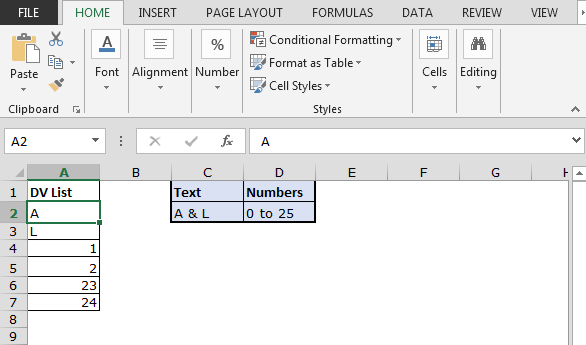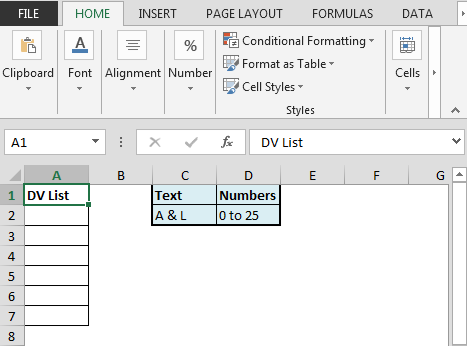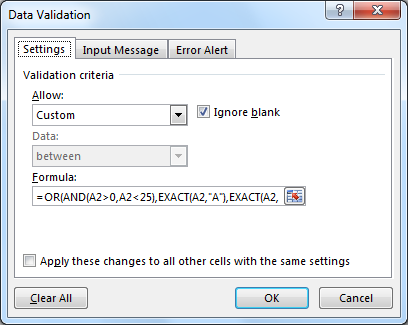# User To Allow Enter Text or Numeric OnlyIn this article, we will learn How to use data validation with Text or Numeric Only in Excel.

Scenario:

Data validation tool restricts the user to input data to ensure its accuracy and consistency in Excel. It helps in the consistency of the data. It guides the user to input the type of option which is necessary for your worksheet. Here the problem is, we need to check the cell, if it has given text and numbers only. If the cell has any punctuation mark, that value must be rejected by Excel. If you want to learn more about Data validation in Excel, follow the link. Here we will just consider the problem, allow Enter Text or Numeric Only.

How to solve the problem?

Here we have a task that only allows entries with no punctuation. So we need to focus  where User To Allow Enter Text or Numeric Only

The use of functions and a tool will help us get through the problem.

1. Data validation tool
2. OR function
3. AND function
4. EXACT function

The formula be used in Data Validation tool. The formula checks the condition and returns True or False. Here the True means that the punctuation doesn't exist, so the tool accepts the value.

Generic formula:

 =OR(AND(cell>0,cell<25),EXACT(cell,"A"),EXACT(cell,"L"))

Explanation:

1. AND function checks the cell value condition for numbers i.e. number between 0 and 25.
2. EXACT function checks the cell value condition for text values i.e A & L.
3. OR function checks if any of the condition is True it returns TRUE.
4. Data validation allows values where the formula returns True.

User To Allow Enter Text or Numeric Only (Excel Forum link)

Question:-

I want to restrict the data entry in range A2:A7 to enter the following:

1. Numbers greater than 0 & less than 25 i.e. (1 to 24)
2. Letters: A & L (not even a & l in small letters)

In short user can enter any value meeting the above two conditions and no other value is allowed in this range.

We can use OR, AND, EXACT functions to get the output.

Following is the snapshot of data:We need to follow the below steps:

• Select range A2:A7
• Press ALT + D + L
• From Settings tab click on Allow drop down & select Custom
• In Formula box enter the formula as =OR(AND(A2>0,A2<25),EXACT(A2,"A"),EXACT(A2,"L"))• Range A2:A7 will accept only the letters either ‘A’ or ‘L’ & numbers between ‘0 to 25’
•• Data validation will pop up error if input is not matching the specified criteria.

In this way we can allow user to enter text or numeric only meeting specific criterias.

Here are all the observational notes regarding using the formula.

Notes:

1. Apply the data validation tool to restrict the user to input invalid value, which doesn't satisfy the condition.
2. Data validation tool can work with numbers and text both.
3. AND, EXACT and OR function returns True and False values as result.
4. Operators like equals to ( = ), less than equal to ( <= ), greater than ( > ) or not equals to ( <> ) can be performed within function applied with numbers only.

Hope this article about How to use data validation with no punctuation in Excel is explanatory. Find more articles on Date & Time functions here. If you liked our blogs, share it with your friends on Facebook. And also you can follow us on Twitter and Facebook. We would love to hear from you, do let us know how we can improve, complement or innovate our work and make it better for you. Write us at info@exceltip.com

Related Articles:

Data Validation in Excel : restricts user to input value manually using this tool in Excel.

Way to use Vlookup function in Data Validation : allow values from the vlookup table in Excel.

Restrict Dates using Data Validation : allow dates in the cell which lays within Excel date format in Excel.

How to give the error messages in Data Validation : customize your data validation cell with error messages in Excel.

Create Drop Down Lists in Excel using Data Validation : restricts users to input data manually in Excel.

Popular Articles:

50 Excel Shortcut to Increase Your Productivity : Get faster at your task. These 50 shortcuts will make you work even faster on Excel.

How to use the IF Function in Excel : The IF statement in Excel checks the condition and returns a specific value if the condition is TRUE or returns another specific value if FALSE.

How to use the VLOOKUP Function in Excel : This is one of the most used and popular functions of excel that is used to lookup value from different ranges and sheets.

How to use the SUMIF Function in Excel : This is another dashboard essential function. This helps you sum up values on specific conditions.

How to use the COUNTIF Function in Excel : Count values with conditions using this amazing function. You don't need to filter your data to count specific values. Countif function is essential to prepare your dashboard.

Terms and Conditions of use

The applications/code on this site are distributed as is and without warranties or liability. In no event shall the owner of the copyrights, or the authors of the applications/code be liable for any loss of profit, any problems or any damage resulting from the use or evaluation of the applications/code.